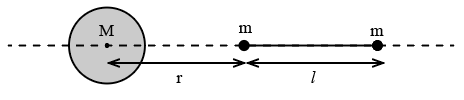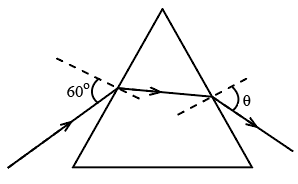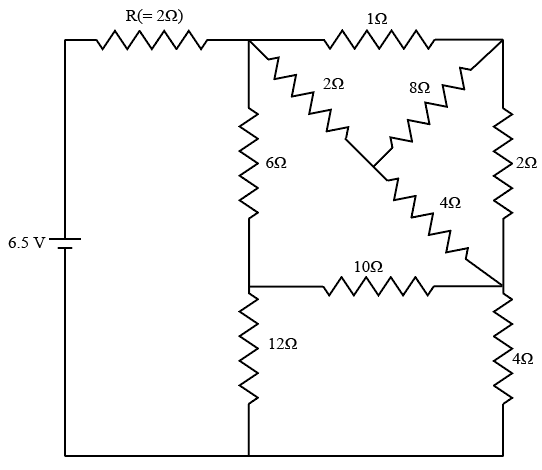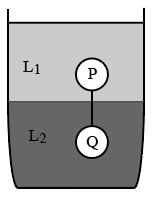Instructions

For the following questions answer them individually

Question 1

# A large spherical mass M is fixed at one position and two identical point masses m are kept on a line passing through the centre of M (see figure). The point masses are connected by a rigid massless rod of length / and this assembly is free to move along the line connecting them. All three masses interact only through their mutual gravitational interaction. When the point mass nearer to M is at a distance r = 3l from M, the tension in the rod is zero for $$m = k \left(\frac {M}{288}\right)$$. The value of k isBackspace
789
456
123
0.-
Clear AllQuestion 2

# The energy of a system as a function of time t is given as $$E(t)= A^2 exp(-\alpha t),$$ where $$\alpha = 0.2 s^{-1}.$$ The measurement of A has an error of 1.25%. If the error in the measurement of time is 1.50%, the percentage error in the value of E(t) at t = 5 s is

Backspace
789
456
123
0.-
Clear AllQuestion 3

# The densities of two solid spheres A and B of the same radii R vary with radial distance r as $$\rho_A(r) = k \left(\frac{r}{R}\right)$$ and $$\rho_B(r) = k \left(\frac{r}{R}\right)^5,$$ respectively, where k is a constant. The moments of inertia of the individual spheres about axes passing through their centres are $$I_A$$ and $$I_B,$$ respectively. If $$\frac{I_B}{I_A} = \frac{n}{10},$$ the value of n is

Backspace
789
456
123
0.-
Clear AllQuestion 4

# Four harmonic waves of equal frequencies and equalintensities $$I_0$$ have phase angles $$0, \frac{\pi}{3}, \frac{2\pi}{3}$$ and $$\pi$$. When they are superposed, the intensity of the resulting wave is $$nI_0.$$ The value of n is

Backspace
789
456
123
0.-
Clear AllQuestion 5

# For a radioactive material, its activity A and rate of change of its activity R are defined as $$A = - \frac{dN}{dt}$$ and $$R = - \frac{dA}{dt},$$ where N(t) is the number of nuclei at time t. Two radioactive sources P (mean life $$\tau$$) and Q (mean life $$2\tau$$) have the same activity at t = 0. Their rates of change of activities at $$t = 2\tau$$ are $$R_P$$ and $$R_Q$$, respectively. If $$\frac{R_P}{R_Q} = \frac{n}{e},$$ then the value of n is

Backspace
789
456
123
0.-
Clear AllQuestion 6

# A monochromatic beam of light is incident at $$60^\circ$$ on one face of an equilateral prism of refractive index n and emerges from the opposite face making an angle $$\theta(n)$$ with the normal (see the figure). For $$n = \sqrt 3$$ the value of $$\theta$$ is $$60^\circ$$ and $$\frac{d \theta}{dn} = m$$. The value of m isBackspace
789
456
123
0.-
Clear AllQuestion 7

# In the following circuit, the current through the resistor $$R (= 2Ω)$$ is I Amperes. The value of I isBackspace
789
456
123
0.-
Clear AllQuestion 8

# An electron in an excited state of $$Li^{2+}$$ion has angular momentum $$\frac{3h}{2\pi}.$$ The de Broglie wavelength of the electron in this state is $$p \pi a_0$$ (where $$a_0$$ is the Bohr radius). The value of p is

Backspace
789
456
123
0.-
Clear AllQuestion 9

# Two spheres P and Q of equal radii have densities $$\rho_1$$ and $$\rho_2$$ respectively. The spheres are connected by a massless string and placed in liquids $$L_1$$ and $$L_2$$ of densities $$\sigma_1$$ and $$\sigma_2$$ and viscosities $$\eta_1$$ and $$\eta_2$$ respectively. They float in equilibrium with the sphere P in $$L_1$$ and sphere Q in $$L_2$$ and the string being taut (see figure). If sphere P alone in $$L_2$$ has terminal velocity $$\overrightarrow{V}_P$$ and Q alone in $$L_1$$ has terminal velocity $$\overrightarrow{V}_Q$$, thenQuestion 10

# In terms of potential difference V, electric current I, permittivity $$\epsilon_0$$, permeability $$\mu_0$$ and speed of light c, the dimensionally correct equation(s) is(are)OR